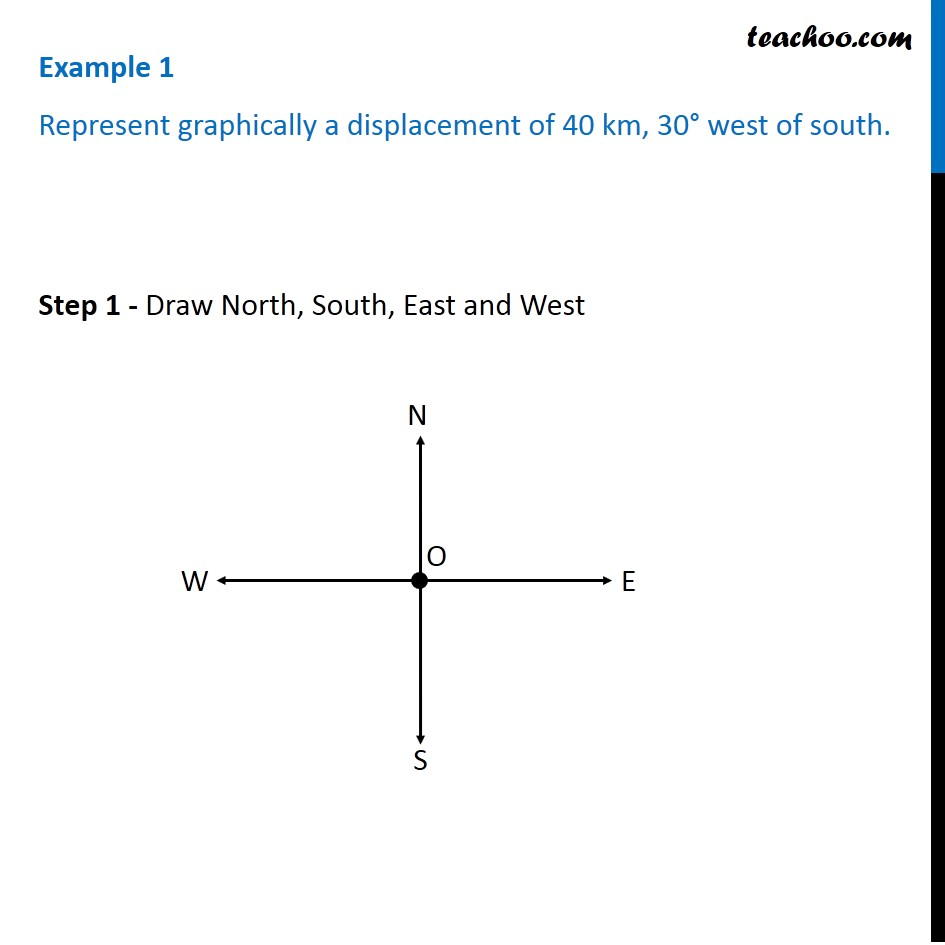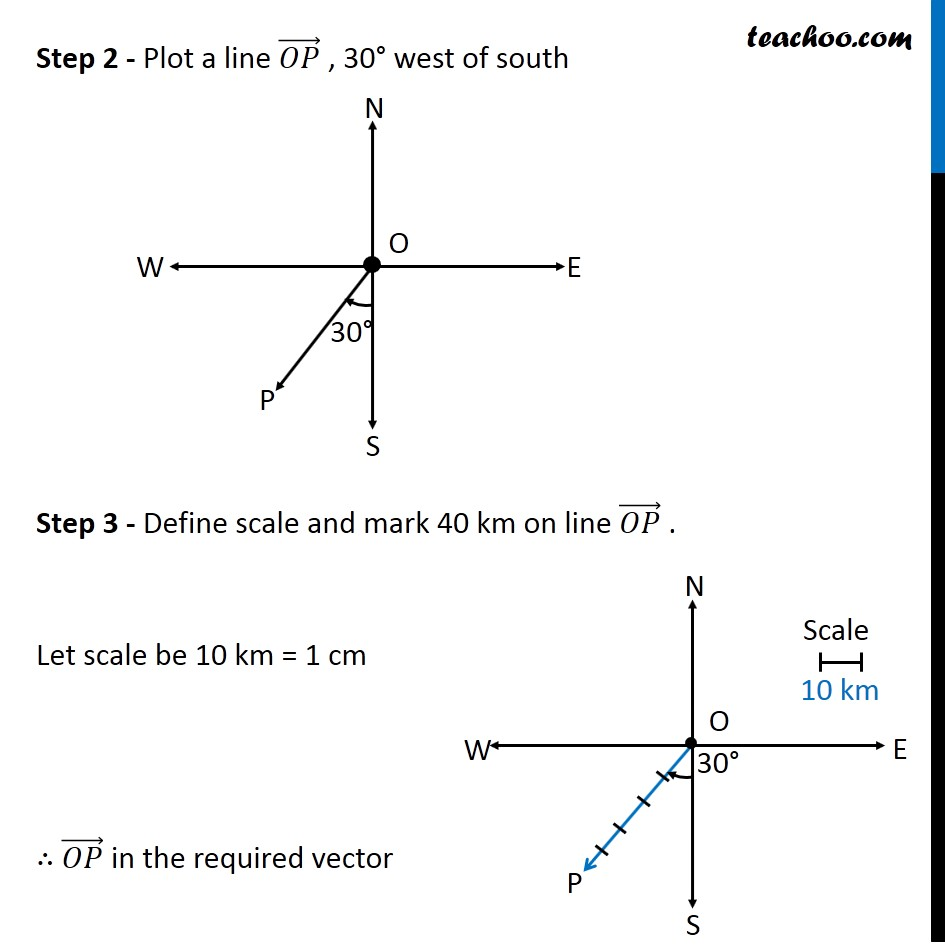Subscribe to our Youtube Channel - https://you.tube/teachoo

1. Chapter 10 Class 12 Vector Algebra
2. Serial order wise
3. Examples

Transcript

Example 1 Represent graphically a displacement of 40 km, 30° west of south. Step 1 - Draw North, South, East and West Step 2 - Plot a line (𝑂𝑃) ⃗ , 30° west of south Step 3 - Define scale and mark 40 km on line (𝑂𝑃) ⃗ . Let scale be 10 km = 1 cm ∴ (𝑂𝑃) ⃗ in the required vector Example 2 Classify the following measures as scalars and vectors. (i) 5 secondsScalar : A quantity which has only magnitude, eg : 10 km Vector: A quantity which has a magnitude as well as a direction Eg : 10 km towards north 5 seconds is a time period. It has only magnitude i.e. 5 and no direction. So, 5 seconds is scalar

Examples